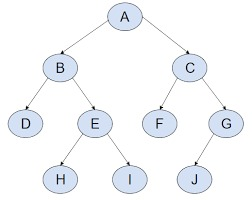# Depth-First SearchAnonymous contributorAnonymous contributor
Published Aug 16, 2023
Contribute to Docs

Depth-first search (DFS) is a traversing algorithm for unweighted graphs.Like BFS (Breadth-first search), it is a foundational algorithm in graph theory from which many other algorithms begin.

## Features

Some of the features and constraints that define the use and functionality of the DFS algorithm include the following:

• DFS traverses a graph by following a given path as far as possible. Once a dead end is reached, the algorithm backtracks to explore the most recent unvisited neighbours. A stack is used to retrieve the next vertex, to restart a search, when a dead end is found in any given iteration.
• It is a relatively simple algorithm that can be built upon for finding cycles within a graph, to conduct a topological sort of vertices, as well as a range of other useful applications.
• It has a time complexity of O(|V| + |E|), which is the sum of the vertices and edges.
• It finds a solution, a path between vertices, but it may or may not be optimal one as in BFS.
• A disadvantage of DFS is that it may get stuck in infinite loop if the depth is infinite.

## Implementation

The example below walks through a basic implementation of DFS that will take a starting node, an end node, and a graph as arguments. The algorithm will return the path it navigated between starting and end node provided.

The DFS algorithm starts with the initial node of graph `G` and goes deeper until it finds the goal node or a node with no children. Because of the recursive nature of the traversal process, a stack data structure can be used to implement the DFS algorithm.

The implementation below can be broken down into the following steps:

1. First, initiate a stack with the starting vertex for the traversal.
2. Pop from the stack and set this vertex as the “current” element or node.
3. Now, find the neighboring vertexes (of the current node), and if they haven’t been visited push them into the stack.
4. If no unvisited vertexes remain, go back and pop a vertex from the stack.
5. Repeat steps 2, 3, and 4 until the stack is empty.

## ExampleThe following is an implementation in Python:

```# The visited variable keeps a record of the nodes exploreddef dfs(graph,start,goal,stack,visited):    stack.append(start)    visited.append(start)    print('The path traversed is:')    while stack:        # Pop from stack to set current element        element=stack.pop()        print(element,end=" ")        if(element==goal):            break        for neighbor in graph[element]:            if neighbor not in visited:                stack.append(neighbor)                visited.append(neighbor)# A dictionary representing the illustrated graphgraph={    'A':['C','B'],    'B':['E','D'],    'C':['G','F'],    'D':[],    'E':['I','H'],    'F':[],    'G':['J'],    'H':[],    'I':[],    'J':[]}start='A'goal='J'visited=[]stack=[]
dfs(graph,start,goal,visited,stack)
```
```The path traversed is:A B D E H I C F G J
```